刺激を受けた:
•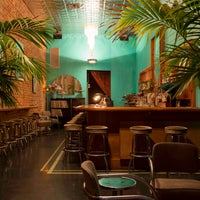•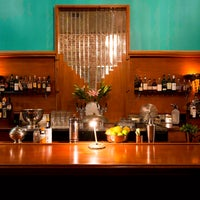•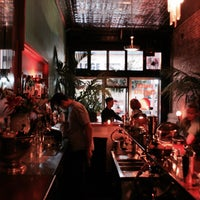••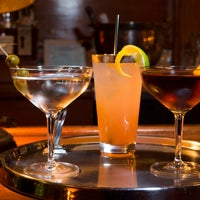•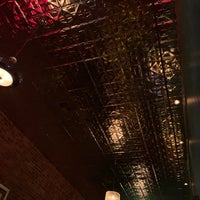•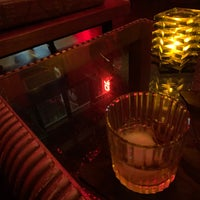•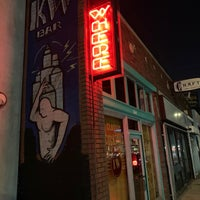•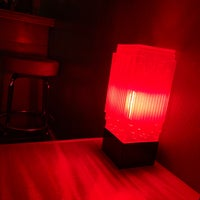•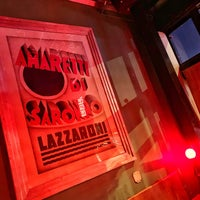•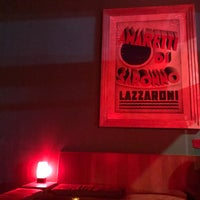•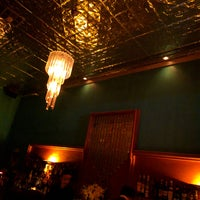•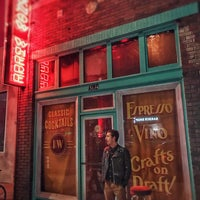•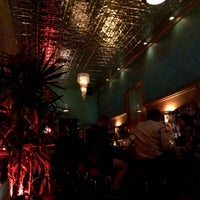•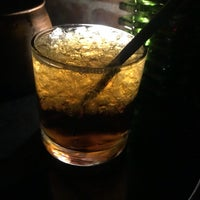•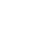34枚の写真をすべて見る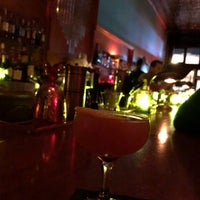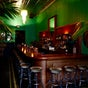# The Know Where Bar

（現在は閉鎖）
バーもぐり酒場\$\$\$\$
East Hollywood, ロサンゼルス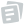7 件のTipとレビュー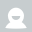ここにTipを残すにはログインしてください。
並べ替え：
• 人気
• 最近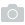34枚の写真

#### 関連する検索

• the know where bar ロサンゼルス •
• the know where bar ロサンゼルス photos •
• the know where bar ロサンゼルス location •
• the know where bar ロサンゼルス address •
• the know where bar ロサンゼルス •
• know where ロサンゼルス •
• know where bar ロサンゼルス •
• know where {bar} ロサンゼルス •
• the know where ロサンゼルス •
• the know where bar ロサンゼルス •
• the know where {bar} ロサンゼルス •
• the know where bar east hollywood ロサンゼルス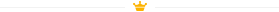Foursquare © 2023  愛情を込めてNYC、CHI、SEA & LAで作られています